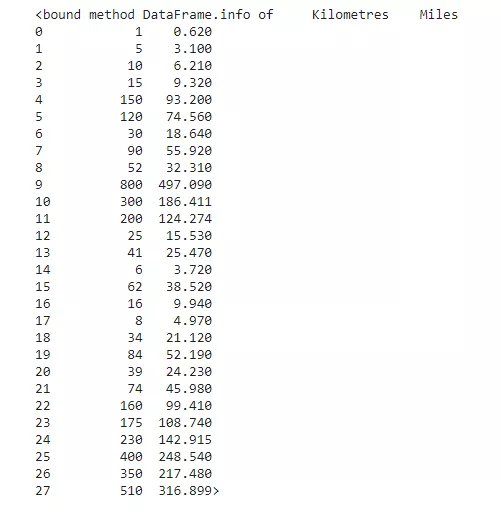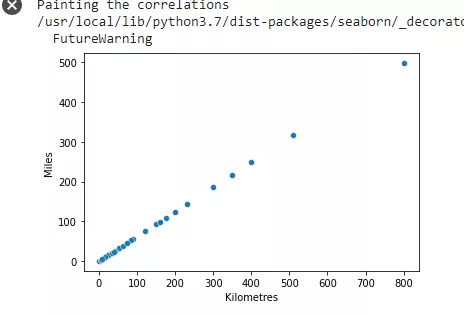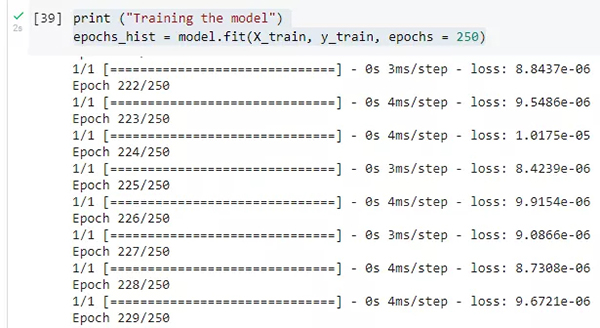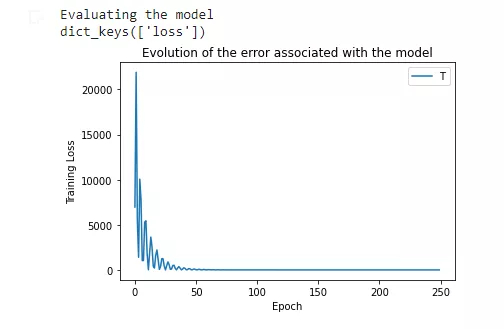# 如何使用TensorFlow构建机器学习模型

TensorFlow 是一个由谷歌开发的库，并在 2015 年开源，它能使构建和训练机器学习模型变得简单。

import tensorflow as tf  import pandas as pd  import seaborn as sns  import matplotlib.pyplot as plt   我们将导入 TensorFlow 来创建我们的机器学习模型。  我们还将导入 Pandas 库来读取包含有公里和英里转换数据的 CSV 文件。  最后，我们将导入 Seaborn 和 Matlotlib 库绘制不同的结果。 加载样例数据print("Painting the correlations")  #Once we load seaborn into the session, everytime a matplotlib plot is executed, seaborn's default customizations are added  sns.scatterplot(df['Kilometres'], df['Miles'])  plt.show()X（公里）是输入，Y（英里）是输出。

print("Define input(X) and output(Y) variables")  X_train=df['Kilometres']  y_train=df['Miles']  创建神经网络

print("Creating the model")  model = tf.keras.Sequential()  model.add(tf.keras.layers.Dense(units=1,input_shape=))  创建神经网络

print("Compiling the model")  model.compile(optimizer=tf.keras.optimizers.Adam(1), loss='mean_squared_error')   训练模型

如果 epoch 的数值越小，误差就会越大；反过来，epoch 的数值越大，则误差就会越小。  如果 epoch 的数值越大，算法的执行速度就会越慢。  print ("Training the model")  epochs_hist = model.fit(X_train, y_train, epochs = 250)print("Evaluating the model")  print(epochs_hist.history.keys())  #graph  plt.plot(epochs_hist.history['loss'])  plt.title('Evolution of the error associated with the model')  plt.xlabel('Epoch')  plt.ylabel('Training Loss')  plt.legend('Training Loss')  plt.show()kilometers = 100  predictedMiles = model.predict([kilometers])  print("The conversion from Kilometres to Miles is as follows: " + str(predictedMiles))

TensorFlow 用于执行此过程的数学非常简单。基本上，本例使用线性回归来创建模型，因为输入变量（公里数）和输出变量（英里数）是线性相关的。在机器学习中，过程中最耗时的部分通常是准备数据。

## 官方微博/微信↑扫描二维码

1.用手机扫左侧二维码；

2.在添加朋友里，搜索关注TechWeb。